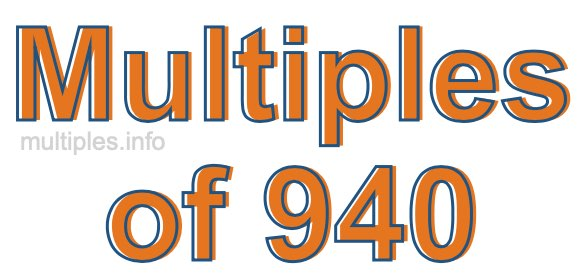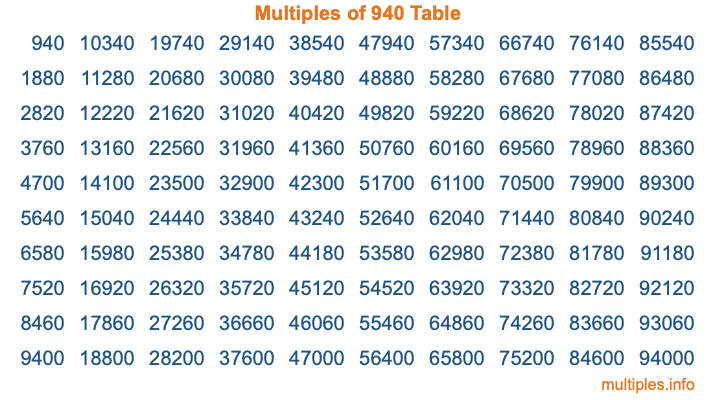Multiples of 940Welcome to the Multiples of 940 page. Here we will first teach you everything you will ever need to know about the multiples of 940, and then give you a study guide summary of everything we taught you to make sure you remember it all. Use this page to look up facts and learn information about the multiples of 940. This page will make you a multiples of nine hundred forty expert!

Definition of Multiples of 940
Multiples of 940 are all the numbers that when divided by 940 equal an integer. Each of the multiples of 940 are called a multiple. A multiple of 940 is created by multiplying 940 by an integer.

Therefore, to create a list of multiples of 940, you start with 1 multiplied by 940, then 2 multiplied by 940, then 3 multiplied by 940, and so on for as long as you want. Thus, the list of the first five multiples of 940 is 940, 1880, 2820, 3760, and 4700. To see a larger list of multiples of 940, see the printable image of Multiples of 940 further down on this page. We also have a category where you can choose any nth multiple of 940.

Multiples of 940 Checker
The Multiples of 940 Checker below checks to see if any number of your choice is a multiple of 940. In other words, it checks to see if there is any number (integer) that when multiplied by 940 will equal your number. To do that, we divide your number by 940. If the the quotient is an integer, then your number is a multiple of 940.

Is  a multiple of 940?

Least Common Multiple of 940 and ...
A Least Common Multiple (LCM) is the lowest multiple that two or more numbers have in common. This is also called the smallest common multiple or lowest common multiple and is useful to know when you are adding our subtracting fractions. Enter one or more numbers below (940 is already entered) to find the LCM.

Check out our LCM Calculator if you need more details about the Least Common Multiple or if you need the LCM for different numbers for adding and subtraction fractions.

nth Multiple of 940
As we stated above, 940 is the first multiple of 940, 1880 is the second multiple of 940, 2820 is the third multiple of 940, and so on. Enter a number below to find the nth multiple of 940.

th multiple of 940

Multiples of 940 vs Factors of 940
940 is a multiple of 940 and a factor of 940, but that is where the similarities end. All postive multiples of 940 are 940 or greater than 940. All positive factors of 940 are 940 or less than 940.

Below is the beginning list of multiples of 940 and the factors of 940 so you can compare:

Multiples of 940: 940, 1880, 2820, 3760, 4700, etc.

Factors of 940: 1, 2, 4, 5, 10, 20, 47, 94, 188, 235, 470, 940

As you can see, the multiples of 940 are all the numbers that you can divide by 940 to get a whole number. The factors of 940, on the other hand, are all the whole numbers that you can multiply by another whole number to get 940.

It's also interesting to note that if a number (x) is a factor of 940, then 940 will also be a multiple of that number (x).

Multiples of 940 vs Divisors of 940
The divisors of 940 are all the integers that 940 can be divided by evenly. Below is a list of the divisors of 940.

Divisors of 940: 1, 2, 4, 5, 10, 20, 47, 94, 188, 235, 470, 940

The interesting thing to note here is that if you take any multiple of 940 and divide it by a divisor of 940, you will see that the quotient is an integer.

Multiples of 940 Table
Below is an image of the first 100 multiples of 940 in a table. The table is in chronological order, column by column. The first column has the first ten multiples of 940, the second column has the next ten multiples of 940, and so on.The Multiples of 940 Table is also referred to as the 940 Times Table or Times Table of 940. You are welcome to print out our table for your studies.

Negative Multiples of 940
Although not often discussed or needed in math, it is worth mentioning that you can make a list of negative multiples of 940 by multiplying 940 by -1, then by -2, then by -3, and so on, to get the following list of negative multiples of 940:

-940, -1880, -2820, -3760, -4700, etc.

Multiples of 940 Summary
Below is a summary of important Multiples of 940 facts that we have discussed on this page. To retain the knowledge on this page, we recommend that you read through the summary and explain to yourself or a study partner why they hold true.

There are an infinite number of multiples of 940.

A multiple of 940 divided by 940 will equal a whole number.

940 divided by a factor of 940 equals a divisor of 940.

The nth multiple of 940 is n times 940.

The largest factor of 940 is equal to the first positive multiple of 940.

940 is a multiple of every factor of 940.

940 is a multiple of 940.

A multiple of 940 divided by a divisor of 940 equals an integer.

940 divided by a divisor of 940 equals a factor of 940.

Any integer times 940 will equal a multiple of 940.

Multiples of a Number
Here you can get the multiples of another number, all with the same attention to detail as we did for multiples of 940 on this page.

Multiples of
Multiples of 941
Did you find our page about multiples of nine hundred forty educational? Do you want more knowledge? Check out the multiples of the next number on our list!